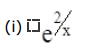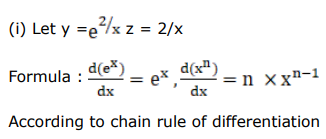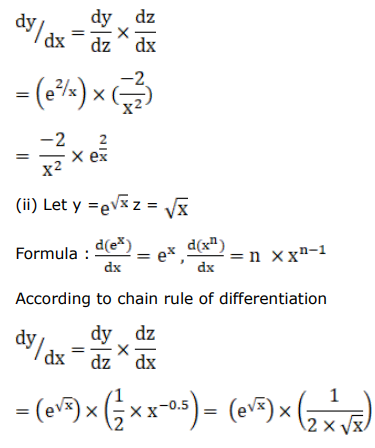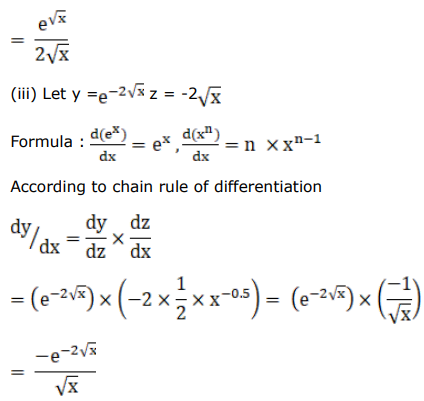# Differentiate each of the following w.r.t.Question:

Differentiate each of the following w.r.t. $x$ :(ii) $e^{\sqrt{x}}$

(iii) $\mathrm{e}^{-2 \sqrt{\mathrm{x}}}$

Solution: